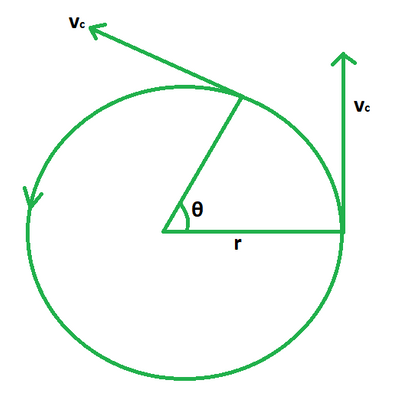# Circular Velocity Formula

• Last Updated : 25 May, 2022

When the velocity changes, the acceleration changes as well, but not in the same direction. As a result, an object moving along a circular path must always have an acceleration that is perpendicular to the velocity. The circular motion can be both uniform and non-uniform. The uniform circular motion formula will be discussed with examples in this topic. Let’s get this topic mastered!

### What is Circular Velocity?

Circular velocity is defined as the velocity possessed by an object while undergoing uniform circular motion. Uniform circular motion is a two-dimensional motion in which an object moves in a circular motion at a constant speed while the velocity changes at each location due to the changing direction of the velocity vector.The circular velocity is directly proportional to the radius of the circular path but inversely proportional to the time taken by the object.

• It is denoted by the symbol vc
• Its unit of measurement is m/s.
• And the dimensional formula is given by [M0L1T-1].

### Circular Velocity Formula

Mathematically, it is defined as the circumference of the circular path divided by the time taken.

vc = 2πr/T

where,

• vc is the circular velocity,
• r is the radius of circular path,
• T is the time taken.

However, the circular velocity can also be calculated when angular velocity ω is given and it is calculated as,

vc = ω r

where,

• ω is the angular velocity,
• r is the radius of the circular path

### Sample Problems

Problem 1: Calculate the circular velocity of an object undergoing circular motion if the radius is 3 m for a time of 4 s.

Solution:

We have,

r = 3

T = 4

Using the formula we have,

vc = 2πr/T

= (2 × 3.14 × 3)/4

= 18.84/4

= 4.71 m/s

Problem 2: Calculate the circular velocity of an object undergoing circular motion if the radius is 5 m for a time of 2 s.

Solution:

We have,

r = 5

T = 2

Using the formula we have,

vc = 2πr/T

= (2 × 3.14 × 5)/2

= 31.4/2

= 15.7 m/s

Problem 3: Calculate the circular velocity of an object undergoing circular motion if radius is 4 m for a time of 7 s.

Solution:

We have,

r = 4

T = 7

Using the formula we have,

vc = 2πr/T

= (2 × 3.14 × 4)/7

= 25.12/7

= 3.58 m/s

Problem 4: Calculate the radius if the circular velocity of an object undergoing circular motion is 5 m/s for a time of 3 s.

Solution:

We have,

vc = 5

T = 3

Using the formula we have,

vc = 2πr/T

=> r = vcT/2π

= (5 × 3)/(2 × 3.14)

= 15/6.28

= 2.38 m

Problem 5: Calculate the radius if the circular velocity of an object undergoing circular motion is 8 m/s for a time of 2 s.

Solution:

We have,

vc = 8

T = 2

Using the formula we have,

vc = 2πr/T

=> r = vcT/2π

= (8 × 2)/(2 × 3.14)

= 16/6.28

= 2.54 m

Problem 6: Calculate the time if the circular velocity of an object undergoing circular motion is 7 m/s for a radius of 5 m.

Solution:

We have,

vc = 7

r = 5

Using the formula we have,

vc = 2πr/T

=> T = 2πr/vc

= (2 × 3.14 × 5)/7

= 31.4/7

= 4.48 s

Problem 7: Calculate the time if the circular velocity of an object undergoing circular motion is 12 m/s for a radius of 8 m.

Solution:

We have,

vc = 12

r = 8

Using the formula we have,

vc = 2πr/T

=> T = 2πr/vc

= (2 × 3.14 × 8)/12

= 50.24/12

= 4.18 s

My Personal Notes arrow_drop_up